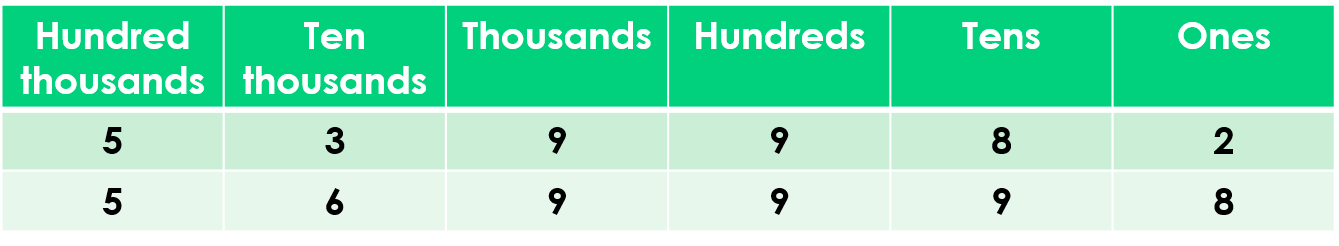1
visibility

Which number is greater?

• A

539,982

• B

569,998

Solution:
Consider the numbers: 539,982 and 569,998.

Notice that both numbers have six digits.

Write these numbers in a place value chart.Observe that the hundred thousands digit in both numbers is the same.

So, we compare the ten thousands digits.

The ten thousands digit in 539,982 is 3 and in 569,998 is 6.

Now, 6 is greater than 3.

So, 569,998 > 539,982

That is, the number 569,998 is greater than 539,982.